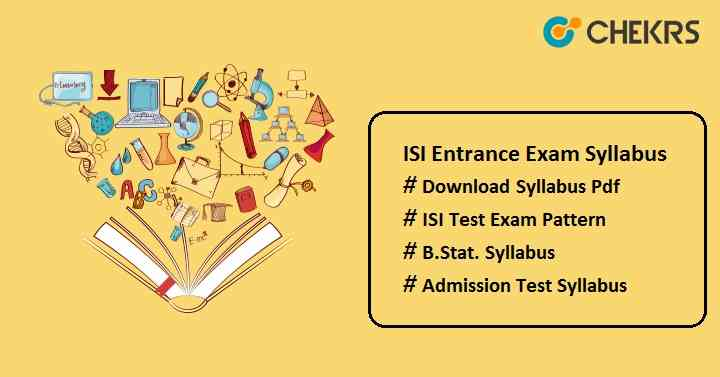# ISI Entrance Exam Syllabus 2023 Pdf – isical.ac.in, Exam Pattern

ISI Entrance Exam Syllabus 2023 Pdf: Indian Statistical Institute (ISI) Kolkata organize an entrance exam at national-level to offer admission to various UG & PG programme. Candidates who will give the ISI admission test to take admission in BStat, BMaths, MTech, PG Diploma programme should check the complete ISI Entrance Exam Syllabus.Candidates can download the complete syllabus in pdf format here and should start their exam preparation to perform well in the ISI Admission Test 2023.

ISI B.Stat Syllabus

Topics Content
Algebra and Number Theory
• Sets, operations on sets.
• Remainder Theorem, Theory of quadratic equations and expressions
• Rational and irrational numbers
• Polynomials: relations between roots and coefficients
• Binomial Theorem
• Permutations and combinations
• Inequalities involving arithmetic, geometric & harmonic means
• Prime numbers, factorization of integers and divisibility
• Logarithms
• Arithmetic and geometric progressions
• Complex numbers.
Trigonometry
• Properties of triangles, heights and distances
• Measures of angles, trignometric and inverse trigonometric functions, trigonometric identities including addition formulae, solutions of trigonometric equations.
Geometry
• Class 10 level plane geometry
• Angle between two lines
• Distance from a point to a line, area of a triangle, equations of a circle, parabola, ellipse and hyperbola and equations of their tangents and normals, mensuration
• Geometry of 2 dimensions with Cartesian and polar coordinates, concept of a locus, equation of a line
Calculus
• Functions – one-one functions, onto functions
• Derivatives and methods of differentiation
• Limit, continuity and differentiability of functions of a single real variable
• Methods of integration, defi
nite and indefi
nite integrals
• The slope of a curve, tangents and normals
• Maxima and minima
• Sequences – bounded sequences, monotone sequences, limit of a sequence
• Use of calculus in sketching graphs of functions
• Evaluation of areas using integrals

To get the complete ISI Admission Test Syllabus of other courses, follow the link provided in the table below.

## ISI Admission 2023 Exam Pattern

Cracking the ISI entrance exam is quite difficult, but candidates can qualify for the ISI admission test by following the ISI Exam Pattern. The exam pattern will help you to know how to attempt paper, how to score highest marks, marking scheme of each paper, total time duration etc.  Candidates are suggested to check the ISI Admission Test Pattern before start exam preparation.

 Mode of Exam Offline Duration of Exam 4 hours (2 hours for each paper.) Type of Questions Multiple Choice Questions & Descriptive Paper No of Paper 2 (Part-1 & Part-2) No of Shift 2 (Morning shift & Afternoon shift) Total Questions 30 questions from Part-1 & 8 questions from Part-2 Total Marks 120 marks for Paper-1 & 80 marks for Paper-2 Marking Scheme +4 for each correct answer (Paper-1) and +10 Marks for each correct response (Paper-2)

We hope the provided ISI Admission Test Syllabus 2023 is helpful for all the candidates who are eligible and applied for the ISI Admission Test. For any other queries, you can contact us by commenting in the comment box given below. We will do our best to help you.  Good Luck!!!

Official Website: ISI Entrance Exam Syllabus 2023 Pdf

#### One Response

1. Vikas kumar Dec 2, 2022
Entrance.Chekrs.com is also part of Chekrs. © 2022 Chekrs.com